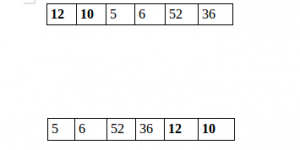Related Articles
Java Program to Split the array and add the first part to the end
• Last Updated : 05 Dec, 2018

There is a given an array and split it from a specified position, and move the first part of array add to the end.Examples:

```Input : arr[] = {12, 10, 5, 6, 52, 36}
k = 2
Output : arr[] = {5, 6, 52, 36, 12, 10}
Explanation : Split from index 2 and first
part {12, 10} add to the end .

Input : arr[] = {3, 1, 2}
k = 1
Output : arr[] = {1, 2, 3}
Explanation : Split from index 1 and first
```

## Recommended: Please try your approach on {IDE} first, before moving on to the solution.

 `// Java program to split array and move first ` `// part to end. ` ` `  `import` `java.util.*; ` `import` `java.lang.*; ` `class` `GFG { ` `    ``public` `static` `void` `splitArr(``int` `arr[], ``int` `n, ``int` `k) ` `    ``{ ` `        ``for` `(``int` `i = ``0``; i < k; i++) { ` ` `  `            ``// Rotate array by 1. ` `            ``int` `x = arr[``0``]; ` `            ``for` `(``int` `j = ``0``; j < n - ``1``; ++j) ` `                ``arr[j] = arr[j + ``1``]; ` `            ``arr[n - ``1``] = x; ` `        ``} ` `    ``} ` ` `  `    ``// Driver code ` `    ``public` `static` `void` `main(String[] args) ` `    ``{ ` `        ``int` `arr[] = { ``12``, ``10``, ``5``, ``6``, ``52``, ``36` `}; ` `        ``int` `n = arr.length; ` `        ``int` `position = ``2``; ` ` `  `        ``splitArr(arr, ``6``, position); ` ` `  `        ``for` `(``int` `i = ``0``; i < n; ++i) ` `            ``System.out.print(arr[i] + ``" "``); ` `    ``} ` `} ` ` `  `// Code Contributed by Mohit Gupta_OMG <(0_o)> `

Output:

```5 6 52 36 12 10
```

Please refer complete article on Split the array and add the first part to the end for more details!

My Personal Notes arrow_drop_up
Recommended Articles
Page :